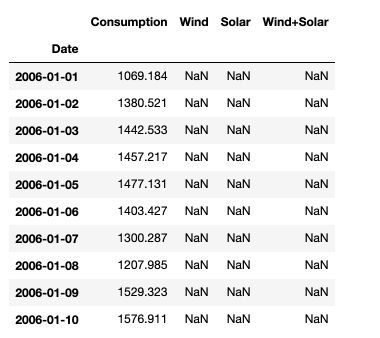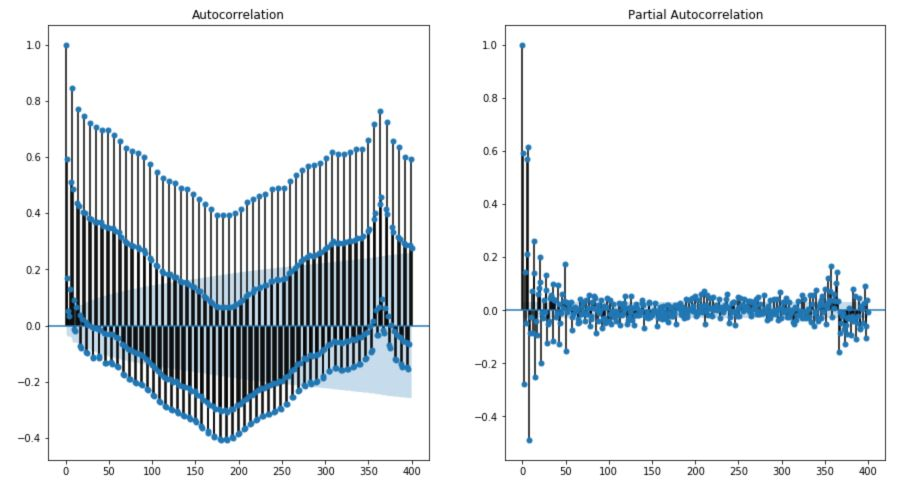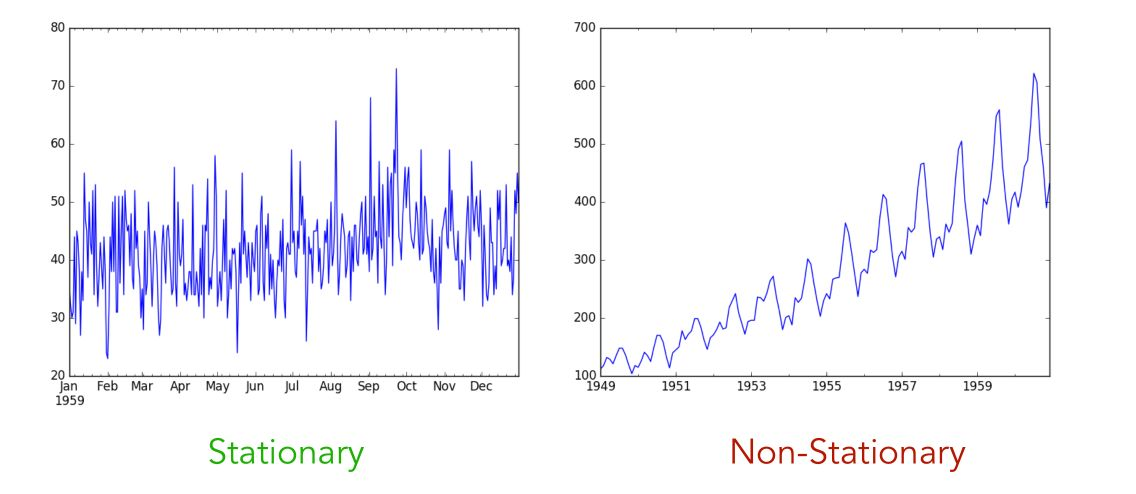In this article, we’ll introduce the key concepts related to time series.

We’ll be using the same data set as in the previous article: Open Power System Data (OPSD) for Germany. The data can be downloaded here

Start by importing the following packages :

### General import
import numpy as np
import pandas as pd
import matplotlib.pyplot as plt
import seaborn as sns
from sklearn import preprocessing
import statsmodels.api as sm

### Time Series
from statsmodels.tsa.ar_model import AR
from sklearn.metrics import mean_squared_error
from pandas.tools.plotting import autocorrelation_plot
from statsmodels.tsa.arima_model import ARIMA
from statsmodels.tsa.seasonal import seasonal_decompose
#from statsmodels.tsa.sarimax_model import SARIMAX

### LSTM Time Series
from keras.models import Sequential
from keras.layers import Dense
from keras.layers import LSTM
from keras.layers import Dropout
from sklearn.preprocessing import MinMaxScaler


df = pd.read_csv('opsd_germany_daily.csv', index_col=0)Then, make sure to transform the dates into datetime format in pandas :

df.index = pd.to_datetime(df.index)


# I. Key concepts and definitions

## 1. Auto-correlation

The auto-correlation $$\rho$$ is defined as the correlation of the series over time, i.e how much the value at time $$t$$ depends on the value at time $$t-j$$ for all $$j$$.

• The auto-correlation $$\rho$$ of order 1 is : $$Corr(y_t, y_{t-1})$$
• The auto-correlation $$\rho$$ of order j is : $$Corr(y_t, y_{t-j})$$
• The auto-covariance $$\rho$$ of order 1 is : $$Cov(y_t, y_{t-1})$$
• The auto-covariance $$\rho$$ of order j is : $$Cov(y_t, y_{t-j})$$

Empirically, the auto-correlation can be estimated by the sample auto-correlation :

$r_j = \frac {Cov^e (y_t, y_{t-j})} {Var^e(y_t)}$

Where : $$Cov^e = \frac {1} {T} \sum_{t-j+1} (y_t - \bar{y_{j+1,T}} ) (y_{t-j} - \bar{y_{1,T-j}})$$

To plot the auto-correlation and the partial auto-correlation, we can use statsmodel package :

fig, axes = plt.subplots(1, 2, figsize=(15,8))

fig = sm.graphics.tsa.plot_acf(df['Consumption'], lags=400, ax=axes)
fig = sm.graphics.tsa.plot_pacf(df['Consumption'], lags=400, ax=axes)We observe a clear trend. The value od consumption at time $$t$$ is negatively correlated with the values 180 days ago, and positively correlated with the values 360 days ago.

## 2. Partial Auto-correlation

The partial autocorrelation function (PACF) gives the partial correlation of a stationary time series with its own lagged values, regressed the values of the time series at all shorter lags. It is a regression of the series against its past lags.

How can we correct auto-correlation ? Take for example :

$y_{t-1} = \beta_0 + \beta_1 X_{t-1} + u_{t-1}$ $y_{t} = \beta_0 + \beta_1 X_{t} + u_{t}$

Therefore, if you substract the first to the second with a coefficient equal to the auto-correlation $$\rho$$ :

$$y_t - \rho y_{t-1} = (1-\rho) \beta_0 + \beta_1 (X_t - \rho X_{t-1}) + e_t$$ for $$t ≥ 2$$

Therefore, if we want to make a regression without auto-correlation :

$\hat{y_{t}} = (1-\rho) \beta_0 + \beta_1 \hat{X_t} + e_t$

Why would we want to remove the auto-correlation?

• to derive the OLS estimator of the parameters $$\beta_1$$ for example
• because there is a bias otherwise since $$u_t$$ would depend on $$u_{t-1}$$

## 3. Stationarity

Stationarity of a time series is a desired property, reached when the joint distribution of $$y_s, y_{s+1}, y_{s+2}...$$ does not depend on $$s$$. In other words, the future and the present should be quite similar. Stationary time series do therefore not have underlying trends or seasonal effect.What kind of events makes a series non-stationary?

• a trend, i.e increasing sales over time
• a seasonality, i.e more sales during the summertime than wintertime

We usually want our series to be stationary even before applying any predictive model!

How can we test if a time series is stationary?

• look at the plots (as above)
• look at summary statistics and box plots as in the previous article. A simple trick is to cut the data set in 2, look at mean and variance for each split, and plot the distribution of values for both splits.
• perform statistical tests, using the (Augmented) Dickey-Fuller test

### Unit roots

Let’s cover into more details the Dickey-Fuller test. To do so, we need to introduce the notion of unit root. A unit root is a stochastic trend in a time series, sometimes called a random walk with drift. If a series has a unit root, it makes it unpredictable due to a systematic pattern.

Let’s consider an autoregressive (we’ll dive deeper later in to this) :

$y_t = a_1 y_{t-1} + a_2 y_{t-2} + ... + a_p y_{t-p} + \epsilon_t$

We define the characteristic equation as :

$$m^p - m^{p-1}a_1 - m^{p-2}a_2 - ... - a_p = 0$$. If $$m = 1$$ is a root to this equation, then the process is said to have a unit root. Equivalently, the process is said to be integrated of order 1 : $$I(1)$$.

In other words, there is a unit root if the previous values keep having a 1:1 impact on the current value. If we consider a simple autoregressive model AR(1) : $$y_t = a_1 y_{t-1} + \epsilon_t$$, the process has a unit root when $$a_1 = 1$$.

If a process has a unit root, then it is non-stationary, i.e the moments of the process depend on $$t$$.

A process is a weakly dependent process, also called integrated of order 0 ( $$I(0)$$ ) if taking the first different of the model is enough to make the series stationary :

$\Delta y_t = y_t - y_{t-1}$

### Dickey-Fuller Test

The Dicker Fuller test is used to assess if a unit root is present in an autoregressive process :

$$H_0 :$$ There is a unit root and the process is not stationary.

$$H_1 :$$ There is no unit root and the process is stationary.

For example, in an AR(1) model where $$y_t = \alpha + \rho y_{t-1} + e_t$$, the hypothesis are :

$H_0 : \rho = 1$ $H_1 : \rho < 1$

The hypothesis $$H_1 > 1$$ would mean an explosive process, and is therefore not considered. When $$\mid \rho \mid < 1$$, then $$Corr(y_t, y_{t-h}) = \rho^h → 0$$.

In practice, we consider the following equation :

$\Delta y_t = \alpha + \theta y_{t-1} + e_t$

We have $$\theta = \rho -1$$ and test $$H_0 : \theta = 0$$.

### Augmented Dickey-Fuller Test

The Augmented Dickey-Fuller Test (ADF) is an augmented version of the Dickey-Fuller test in the sense that it can test for a more complex set of time series models. For example, consider an ADF on an AR(p) process :

$\Delta_t = \alpha + \theta y_{t-1} + \gamma_1 \Delta y_{t-1} + ... + \gamma_p \Delta y_{t-p} + \epsilon_t$

And the null hypothesis : $$H_0 : \theta = 0$$.

## 4. Ergodicity

Ergodicity is the process by which we forget the initial conditions. This is reached when auto-correlation of order $$k$$ tends to $$0$$ as $$k$$ tends to $$\infty$$.

According to the ergodicity theorem, when a time series is strictly stationary and erdogic, and $$E(Y_T) < \infty$$ when $$T → \infty$$, then $$\frac {1} {n} \sum_i y_t → E(Y_T)$$

## 5. Exogeneity

Exogeneity describes the relation between the residuals and the explanatory variables. The exogeneity is said to be strict if :

$$y_t = \beta_0 + \beta_1 X_{t-1} + ... + \beta_k X_{tk} + u_t$$ and $$E(u_t \mid X) = 0$$ for all t.

The exogenity is said to be contemporary when :

$$E(u_t \mid X_{t-1}, ..., X_{t-k}) = E(u_t \mid X_t) = 0$$ which is a weaker assumption, but satisfies the consistency hypothesis.

## 6. Long term effect

Let’s consider again the model : $$y_t = \beta_0 + \beta_1 X_{t-1} + ... + \beta_k X_{tk} + u_t$$. In that case, we can estimate the long term effect as :

$LRP = \beta_0 + \beta_1 + ... + \beta_q$

We can test the Granger causality using a Fisher test :

$$H_0 : \beta_0 = \beta_1 = ... = \beta_q = 0$$. Under this hypothesis, no past value of $$X$$ would allow to predict $$Y$$.

Conclusion : I hope you found this article useful. Don’t hesitate to drop a comment if you have a question.

Categories:

Updated: